Courses

# Test: Equilibrium 1 - From Past 28 Years Questions

## 17 Questions MCQ Test Chemistry Class 11 | Test: Equilibrium 1 - From Past 28 Years Questions

Description
This mock test of Test: Equilibrium 1 - From Past 28 Years Questions for NEET helps you for every NEET entrance exam. This contains 17 Multiple Choice Questions for NEET Test: Equilibrium 1 - From Past 28 Years Questions (mcq) to study with solutions a complete question bank. The solved questions answers in this Test: Equilibrium 1 - From Past 28 Years Questions quiz give you a good mix of easy questions and tough questions. NEET students definitely take this Test: Equilibrium 1 - From Past 28 Years Questions exercise for a better result in the exam. You can find other Test: Equilibrium 1 - From Past 28 Years Questions extra questions, long questions & short questions for NEET on EduRev as well by searching above.
QUESTION: 1

### In a buffer solution containing equal concentration of B– and HB, the Kb for B– is 10–10. The pH of buffer solution is : 

Solution: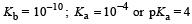For the buffer solution containing equal concentration of B and HB
pH = pKa + log 1
pH = pKa = 4
The octahedral complex ion [ CoCl2(NH3)4]+ i.e., tetra amminedichloro cobalt (III) ion exists as cis and trans isomers.

QUESTION: 2

### Which one of the following molecular hydrides acts as a Lewis acid? 

Solution:

Boron in B2H6 is electron deficient

QUESTION: 3

### The reaction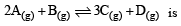begun with the concentrations of A and B both at an initial value of 1.00 M. When equilibrium is reached, the concentration of D is measured and found to be 0.25 M. The value for the equilibrium constant for this reaction is given by the expression 

Solution: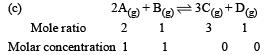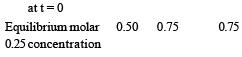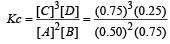QUESTION: 4

A buffer solution is prepared in which the concentration of NH3 is 0.30M and the concentration of NH4+ is 0.20 M. If the equilibrium constant, Kb for NH3 equals 1.8 × 10–5, what is the pH of this solution ? (log 2.7 = 0.433). 

Solution:

Given [NH3] = 0.3 M, [NH4+] = 0.2 M, Kb = 1.8 × 10–5.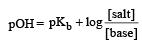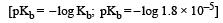∴  pKb = 4.74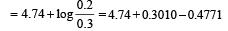= 4.56
pH = 14 – 4.56 = 9.436

QUESTION: 5

Which of the following is least likely to behave as Lewis base ? 

Solution:

BF3 behaves as lewis acid.

QUESTION: 6

In qualitative analysis, the metals of Group I can be separated from other ions by precipitating them as chloride salts. A solution initially contains Ag+ and Pb2+ at a concentration of 0.10 M. Aqueous HCl is added to this solution until the Cl concentration is 0.10 M. What will the concentrations of Ag+ and Pb2+ be at equilibrium?
(Ksp for AgCl = 1.8 × 10–10,
Ksp for PbCl2 = 1.7 × 10–5) [2011M]

Solution:

Ksp = [Ag+] [Cl] 1.8 × 10–10 = [Ag+] [0.1] [Ag+] = 1.8 × 10–9 M
Ksp = [Pb+2] [Cl]2 1.7 × 10–5 = [Pb+2] [0.1]2 [Pb+2] = 1.7 × 10–3 M

QUESTION: 7

pH of a saturated solution of Ba(OH)2  is 12. The value of solubility product (KSP)of Ba(OH)2  is : 

Solution:

Given   pH = 12 or  [H+] = 10–12
Since, [H+] [OH] = 10–14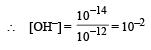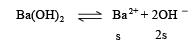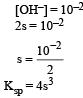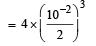= 5 × 10–7

QUESTION: 8

Equimolar solutions of the following substances were prepared separately. Which one of these will record the highest pH value ? 

Solution:

(AlCl3, LiCl & BeCl2) ) all these solutions are acidic due to cationic hydrolysis, where as BaCl2, is salt of strong base and strong acid, hence its solution will almost neutral i.e., pH ≈ 7.

QUESTION: 9

Buffer solutions have con stant acidity and alkalinity because 

Solution:

Lets take an example of an acidic buffer CH3COOH and CH3COONa.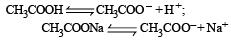when few drops of HCl are added to this buffer, the H+ of HCl immediatly combine with CH3COO  ions to form undissociated acetic acid molecules. Thus there will be no appreciable change in its pH value. Like wise if few drops of NAOH are added, the OH – ions will combine with H+ ions to form unionised water molecule. Thus pH of solution will remain constant.

QUESTION: 10

Given that the equilibrium constant for the reaction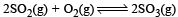has a value of 278 at a particular temperature. What is the value of the equilibrium constant for the following reaction at the same temperature ?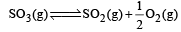[2012 M]

Solution: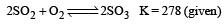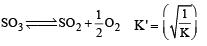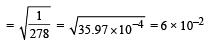QUESTION: 11

Given the reaction between 2 gases represented by A2 and B2 to give the compound AB(g).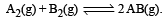At equilibrium, the concentration
of A2 = 3.0 × 10–3 M
of B2= 4.2 × 10–3 M
of AB = 2.8 × 10–3 M
lf the reaction takes place in a sealed vessel at 527°C, then the value of KC will be : [2012 M]

Solution: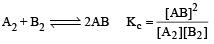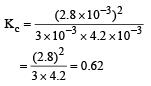QUESTION: 12

Identify the correct order of solubility in aqueous medium:    [NEET 2013]

Solution:

Solubility of alkali metal is maximum among the following. Among ZnS (1.7 × 10–5) & CuS (8 × 10–37) ZnS has higher value  of Ksp.

QUESTION: 13

Which of these is least likely to act as Lewis base?    [NEET 2013]

Solution:

BF3 is Lewis acid(e pair acceptor)

QUESTION: 14

The values of Ksp of CaCO3 and CaC2O4 are 4.7 × 10–9 and 1.3 × 10–9 respectively at 25°C. If the mixture of these two is washed with water, what is the concentration of Ca2+ ions in water?     [NEET Kar. 2013]

Solution: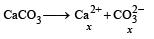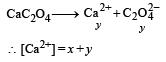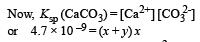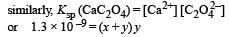On solving, we get [Ca2+] = 7.746 × 10–5 M

QUESTION: 15

At 100°C the Kw of water is 55 times its value at 25°C. What will be the pH of neutral solution? (log 55 = 1.74) [NEET Kar. 2013]

Solution:

Kw at  25°C = 1 × 10–14 At 25ºC

Kw = [H+] [OH] = 10–14 At 100°C (given) Kw = [H+] [OH] = 55 × 10–14
∵ for a neutral solution [H+] = [OH]
∴ [H+]2 = 55 × 10–14
or [H+] = (55 × 10–14)1/2
∵  pH = – log [H+]
On taking log on both side – log [H+] = –log (55 × 10–14)1/2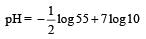pH =  – 0.87 + 7
=  6.13

QUESTION: 16

Th e dissociation constan t of a weak acid is 1 × 10– 4. In order to prepare a buffer solution with a pH = 5 the [Salt]/[Acid] ratio should be [NEET Kar. 2013]

Solution: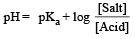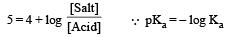Given  Ka =  1 × 10– 4
∴ pKa =  – log (1× 10– 4) =  4
Now from Handerson equation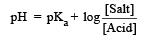Putting the values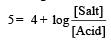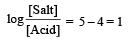Taking antilog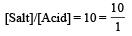QUESTION: 17

Accumulation of lactic acid (HC3H5O3), a monobasic acid in tissues leads to pain and a feeling of fatigue. In a 0.10 M aqueous solution, lactic acid is 3.7% dissociated. The value of dissociation constant, Ka, for this acid will be: [NEET Kar. 2013]

Solution: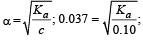Ka = (0.037)2 x 0.10 = 1.37 ×10–4